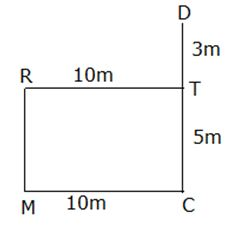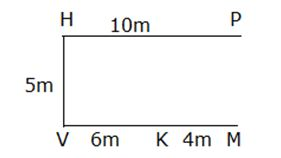# IBPS Clerk Mains Reasoning Questions 2019 (Day-13) High Level-New Pattern

Dear Aspirants, Reasoning Ability plays a crucial role in Banking and all other competitive exams. To enrich your preparation, here we have provided New Pattern Reasoning Questions for IBPS Clerk Mains. Candidates those who are going to appear in IBPS Clerk Mains can practice these questions daily and make your preparation effective.

[WpProQuiz 4869]

(Directions 1–4): A string of numbers is given as input. The further steps given are obtained by applying certain logic. Each step is a resultant of previous step only. Study the following information carefully and answer the questions given below it.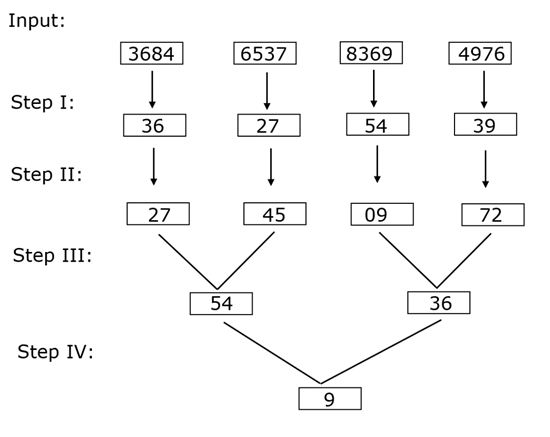As per above applied logic in above steps, find appropriate step for given input: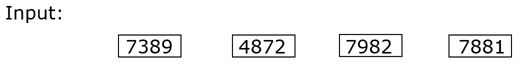1) Who is the difference of highest to lowest number in step II?

a) 33

b) 9

c) 48

d) 24

e) None of these

2) Which of the following will be the output in step IV?

a) 12

b) 23

c) 21

d) 19

e) None of these

3) What will be resultant when highest number in step III is divided by lowest number is same step?

a) 24

b) 28

c) 32

d) 18

e) None of these

4) What is the remained when highest number in step I is divided by lowest number in step II?

a) 9

b) 3

c) 13

d) 15

e) None of these

(Directions 5–8): There are five 4-digit numbers as given below. Answer the following questions based on these numbers.

6284 7824 2963 4825 9528

5) For how many 4-digit number sum of product of first two digit and last two digit is a perfect square?

a) Four

b) Two

c) One

d) Three

e) None

6) Digits within 4-digit number are arranged in ascending order from left to right then, what is product of 2nd digit of second lowest number and 3rd digit of second highest number after rearrangement?

a) 24

b) 42

c) 28

d) 35

e) None of these

7) If 1st and 4th& 2nd and 3rd digit of each 4-digit number are interchanged then what is difference of second lowest to third lowest number after rearrangement?

a) 539

b) 595

c) 458

d) 1134

e) None of these

8) If each 4-digit number is divided by its highest digit then how many such 4-digit numbers are possible for which remainder is less than 4?

a) One

b) Three

c) Four

d) Two

e) None

Directions (9-10): Study the following information carefully and answer the questions given below:

If,

‘Q#^S’ means Point Q is 8m north of Point S.

‘Q\$%S’ means Point Q is 10m east of Point S.

‘Q^&S’ means Point Q is 4m west of Point S.

[email protected]*S’ means Point Q is 5m south of Point S.

9) If D#^C\$%[email protected]*R, then how far and in which direction Point R with respect to Point T, if Point T is 3m south of Point D?

a) 5m, West

b) 3m, West

c) 8m, West

d) 10m, West

e) None of these

10) If K^&M\$%[email protected]*H, P\$%H, then how far and in which direction Point P with respect to Point M?

a) 5m, South

b) 5m, North

c) 7m, North

d) 7m, South

e) None of these

Directions (1-4):

We have: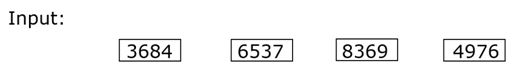Step I: In this step following logic is applied: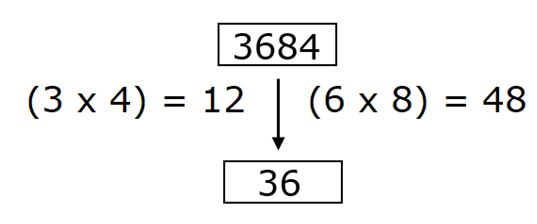Step III: In this step following logic is applied: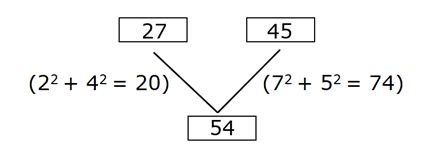Clearly, in step III result can be obtained from difference of results:

Result = (74 – 20) = 54

Step IV: In this step following logic is applied: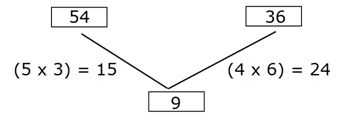Clearly, in step IV result can be determined from difference of results:

Result = (24 – 15) = 9

From above logical steps we get following results for given input: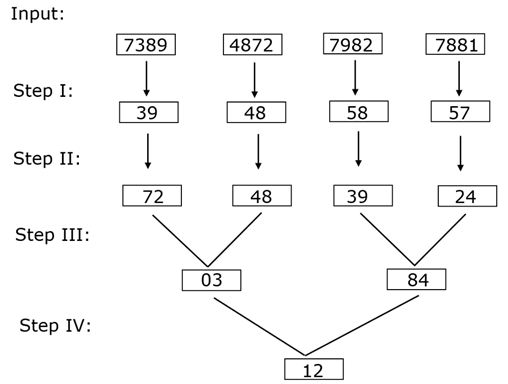Clearly, required difference = (72 – 24) = 48

Hence, option C is correct choice.

Clearly, 12 is the final output in step IV.

Hence, option A is correct choice.

Clearly, required number = 84/3 = 28

Hence, option B is correct choice.

Clearly, required remainder when 58 is divided by 24 à 10

Hence, option E is correct choice.

Directions (5-8):

We have:

6284 7824 2963 4825 9528

Sum of product of 6284 = (6 x 2) + (8 x 4) = 44

Sum of product of 7824 = (7 x 8) + (2 x 4) = 64

Sum of product of 2963 = (2 x 9) + (6 x 3) = 36

Sum of product of 4825 = (4 x 8) + (2 x 5) = 42

Sum of product of 9528 = (9 x 5) + (2 x 8) = 61

Clearly, only two such 4-digit number are possible.

Hence, option B is correct choice.

We have:

6284 7824 2963 4825 9528

After rearranging digits in alphabetical order we have:

2468 2478 2369 2458 2589

2nd digit of second lowest number 2458 à 4

3rd digit of second highest number 2478 à 7

Required product = (4 x 7) = 28

Hence, option C is correct choice.

We have:

6284 7824 2963 4825 9528

After interchanging digits of 4-digit number we have:

4826 4287 3692 5284 8259

Second lowest number = 4287

Third lowest number = 4826

Required difference = (4826 – 4287) = 539

Hence, option A is correct choice.

We have:

6284 7824 2963 4825 9528

After dividing 6284 by 8 we get remainder = 4

After dividing 7824 by 8 we get remainder = 0

After dividing 2963 by 9 we get remainder = 2

After dividing 4825 by 8 we get remainder = 1

After dividing 9528 by 9 we get remainder = 6

Clearly, only three such 4-digit number for which remained is less than 4.

Hence, option B is correct choice.